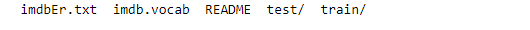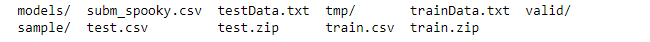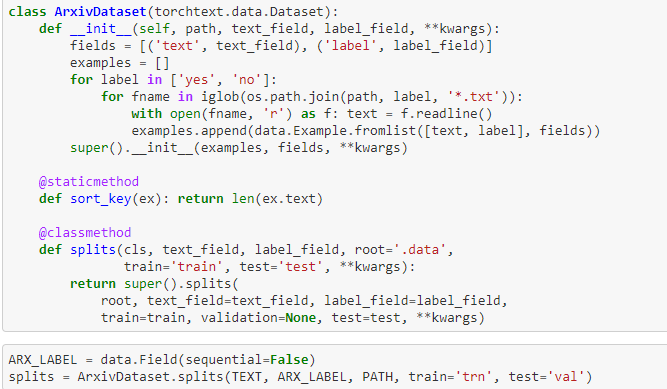# Kaggle Comp: NLP Classification

Can I join as well?

Sure…

I’m trying to follow the steps on the IMDB sample
FILES = dict(train=TRN_PATH, validation=VAL_PATH, test=VAL_PATH)
md = LanguageModelData(PATH, TEXT, **FILES, bs=bs, bptt=bptt, min_freq=10)

## but in our case the information is stored in pandas data frames. This is what I have FILES = dict(train_df=df_train, val_df=df_valid, test_df=test) md = LanguageModelData.from_dataframes(TEXT, trn_ds=df_train, val_ds=df_valid, test_ds=test, bs=bs, bptt=bptt, min_freq=10) But it’s sending error message

TypeError Traceback (most recent call last)
in ()
----> 1 md = LanguageModelData.from_dataframes(TEXT, trn_ds=df_train, val_ds=df_valid, test_ds=test, bs=bs, bptt=bptt, min_freq=10)

TypeError: from_dataframes() missing 3 required positional arguments: ‘col’, ‘train_df’, and ‘val_df’

Could you please review and let me know what I’m doing wrong here?

I’m not sure if there is support for doing this directly through the fastai codebase, but I just looped through and created text files similar to the format of the IMDB data. Is very fast and works perfect.

I figured this out with this code

Code
FILES = dict(train_df=df_train, val_df=df_valid, test_df=test)
md = LanguageModelData.from_dataframes(TEXT, col=‘text’, **FILES, bs=bs, bptt=bptt, min_freq=10)

df_train, df_valid and test are pandas DataFrames

1 Like

`md = LanguageModelData.from_dataframes(TEXT, col=‘text’, **FILES, bs=bs, bptt=bptt, min_freq=10)`

This line doesn’t give you an error? The latest definition in the code is,

`def from_dataframes(cls, path, field, col, train_df, val_df, test_df=None, bs=64, bptt=70, **kwargs):`

So, I think the notebook code needs to call this as,

`md = LanguageModelData.from_dataframes(PATH, TEXT, col=‘text’, **FILES, bs=bs, bptt=bptt, min_freq=10)`

or

`md = LanguageModelData.from_dataframes(PATH, TEXT, col=‘text’, train_df=df_train, val_df=df_valid, test_df=df_test, bs=bs, bptt=bptt, min_freq=10)`

2 Likes

Looks like the last fastai update changed the definition

Is the PATH really needed on the method LanguageModelData.from_dataframes?

Yes the learner needs to know where to store models etc.

if it is not too late, can I also join the group?

Hi,

I am looking for torchtext examples on pre-processing text data and loading pre-trained word embedding matrices. Can someone point me in the right direction?

Thanks!!

### Does dataset class expects data to be in dogscats or Keras style structure e.g. train/all/pos or train/all/neg

IMDBSpooky’s content:Should we convert our data into IMDB type structure or dataset class can be customized to handle csv type data?
My dataset is similar.@jeremy, @yinterian: Could you pls. share your thoughts?

2 Likes

I want to try myself in this competition as well. But I’m worried about training time for language model. In imdb notebook Jeremy did around 60 epochs for language model which would train around 20 hours on p2 Amazon.
Do you guys train your own language models for this competition based on dataset provided by kaggle or is it possible to use other pretrained models ?

1 Like

@rob Did you manage to fix overfitting issue?

It’s fast on p2 (say few minutes to 1 hour). The data is less.

@rob Did you manage to fix overfitting issue?

No, I just trained until training loss became better than validation loss and stopped there

How much loss could you manage to reduce.

Down to 3.2 or so. Note this wasn’t for spooky author, it was for another dataset

interesting.

Is Spooky worth trying with DL? Do we’ve enough data?

1 Like